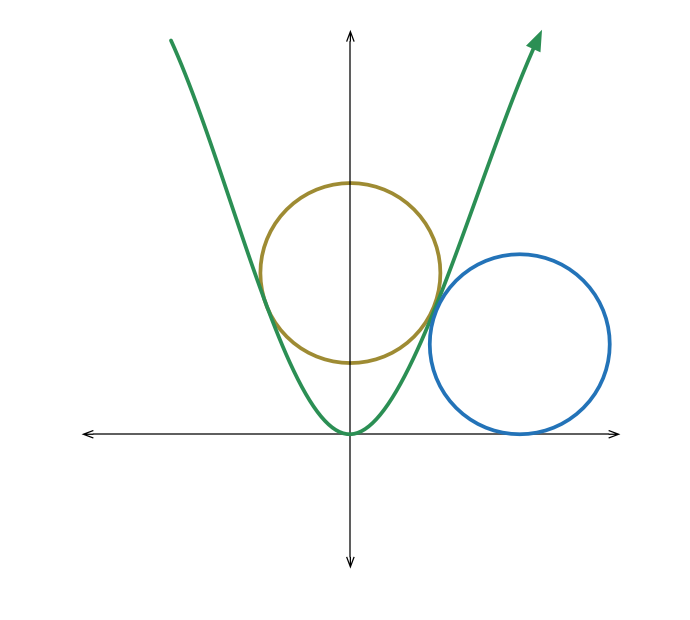# Gently does it

Geometry Level 4A circle of radius $r$ is positioned so that it has two points of tangency with the parabola $y = x^{2}$. Another circle, again of the same radius $r$, is positioned (entirely in the first quadrant) so that it is tangent to both $y = x^{2}$ and the positive $x$-axis.

There is a unique value of $r$ so that the two circles described above, both of radius $r$, are also tangent to one another. If $r = \dfrac{a}{b}$, where $a$ and $b$ are positive coprime integers, then find $a + b$.

×